Statistical and probability models: Represent data effectively

# Unit 2: Bar graphs and histograms

Dylan Busa### Unit 2: Bar graphs and histograms

By the end of this unit you will be able to:

• Construct a bar graph.
• Construct a compound bar graph.
• Construct a histogram.

## What you should know

Before you start this unit, make sure you can:

• Create ungrouped and grouped frequency distributions. Refer to unit 1 in this Subject outcome if you need help with this.

## Introduction

Bar graphs (or charts) and histograms are everywhere. They may even be the most common method of representing data in everyday life. They can be used to represent a wide variety of different kinds of data. They are also easy to create and easy to read.

Here are a few real examples of bar graphs (see Figures 1 and 2) and histograms (see Figures 3 and 4).

At this point, you may be wondering what the differences between a bar graph and histogram are. On the surface they look the same. However, there are some important differences in the way in which they are drawn, and the kind of data they are used to represent.

Have a look at the bar graph and the histogram comparison in Figure 5.

1. In the bar graph, the bars do not touch. This is because bar graphs are used to represent categorical data and are a diagrammatic comparison of discrete variables. Histograms, on the other hand, represent a frequency distribution of continuous numerical variables. Because the data are continuous and there are no gaps in the intervals or classes of the frequency distribution, there can be no gaps between the bars.
2. In the case of a bar graph, it is quite common to rearrange the blocks, from highest to lowest or vice versa. But with a histogram, this cannot be done. The bars must be shown in the same order as the sequence of classes.
3. The width of the blocks in a histogram may or may not be same, while the width of the bars in a bar graph is always same. Generally, the bars of a histogram will be the same width because the width of the bar represents the width of the class and classes are usually the same width.

### Note

For more on the differences between bar graphs and histograms, watch the video called “How a histogram is different than a bar chart?”.

How a histogram is different than a bar chart? (Duration: 01.54)## Simple bar graphs and compound bar graphs

Because bar graphs deal with categorical data, they are easy to draw. In the previous unit, we came across a set of survey data about which flowers a group of ladies favoured. Here is the raw data again.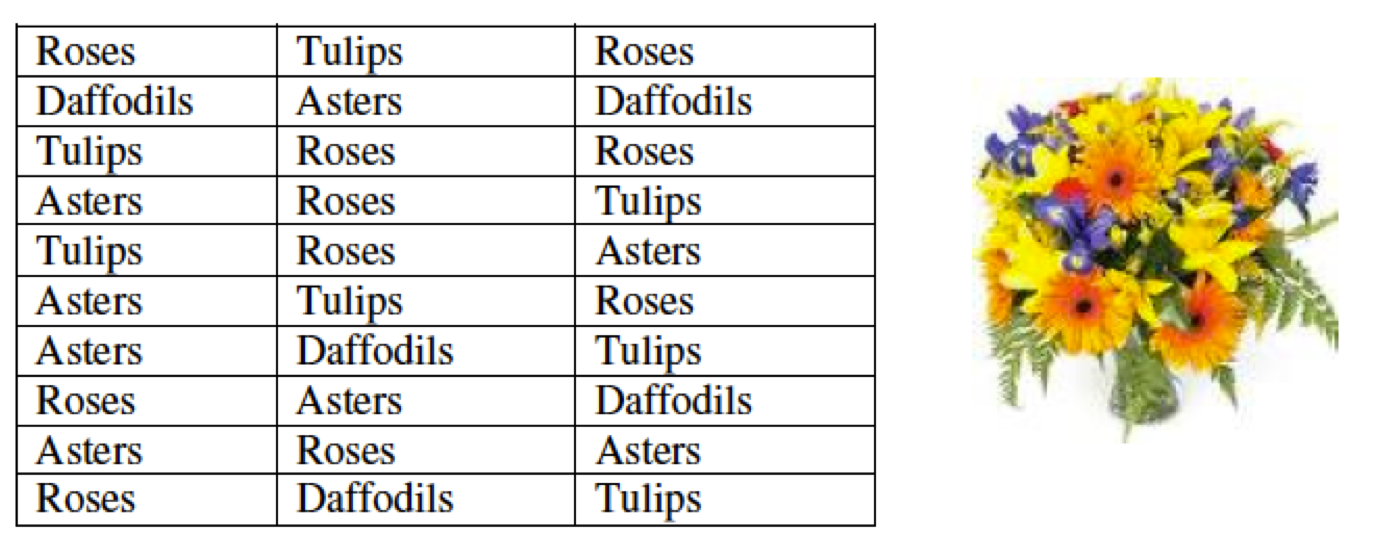The first step in drawing a bar graph is to create an ungrouped frequency distribution. This we completed in the previous unit. The results are shown below in Table 1.Table 1: Frequency distribution of favourite flowers among a group of $\scriptsize 30$ ladies

Next, we draw our axes placing the categories on the x-axis and, in this case, the frequencies on the y-axis. Lastly, we draw in each of the bars to represent the frequency of each category. Remember to always title your bar graphs. The completed bar graph is shown in Figure 6. Labels have been added above each bar to make the graph easier to read, but these are not essential.

A compound bar graph is very similar to an ordinary bar graph except that it represents multiple pieces of information in a single bar. The example in Figure 2 above shows the revenues, expenses and resultant increase in net assets for each of three years. In this case, each category (years) contained three subcategories. The bars representing the sub-categories can be touching but the categories must still be separated by a gap.

Another type of compound bar graph is like that shown in Figure 7. Here, instead of the bars being placed alongside each other, they are stacked on top of each other. It shows the comparative electrical power generation per month in Germany from solar and wind sources.

Whether the bars are placed alongside or stacked is a matter of whether it is more important for the graph to communicate the relative differences within categories or the combined differences between categories.

As with simple bar graphs, compound bar graphs are compiled from the data in frequency distributions. In almost all cases, however, compound bar graphs require a key to help readers understand what the different sub-categories are.

### Note

Watch this excellent summary video on bar graphs called “Bar Graphs”.

Bar Graphs (Duration: 04.05)### Exercise 2.1

GQ Magazine held a poll to determine the favourite DJs of NC(V) learners. The results of the first $\scriptsize 30$ responses are presented in the following frequency distribution. Draw a bar graph to represent this data.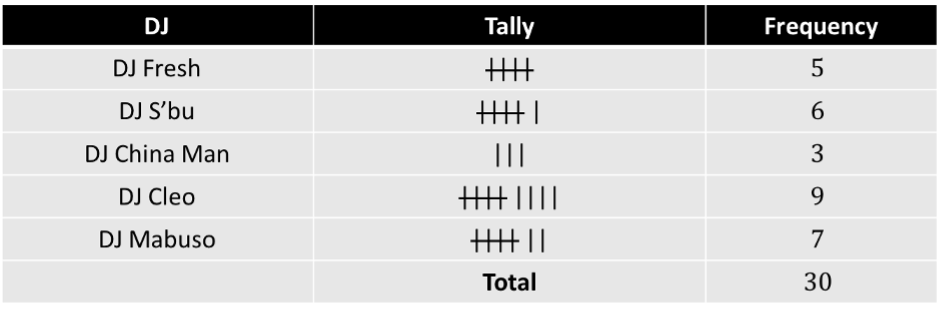The full solutions are at the end of the unit.

## Histograms

As we saw earlier, histograms are used to present grouped numerical data. In the previous unit, we created a grouped frequency distribution of the following data of the heights (in $\scriptsize \text{cm}$) of a group of $\scriptsize 190$ students. The resulting frequency distribution is shown in Table 2.

$\scriptsize 165, 148, 158, 150, 160, 165, 150, 156, 155, 164,~162, 160, 158, 148, 158, 140, 146, 160, 148, 152, 139, 165, 148, 160, 156, 158, 170, 155, 160, 148, 155, 158, 179, 170, 158, 161, 155, 160, 163, 178, 138, 172, 170, 156, 160, 160, 171, 140, 160, 170, 175, 148, 170, 177, 155, 167, 154, 160, 170, 155, 136, 179, 150, 167, 148, 160, 164, 167, 157, 165, 163, 140, 162, 178, 160, 170, 163, 162, 165, 175, 165, 152, 147, 180, 148, 170, 165, 167, 165$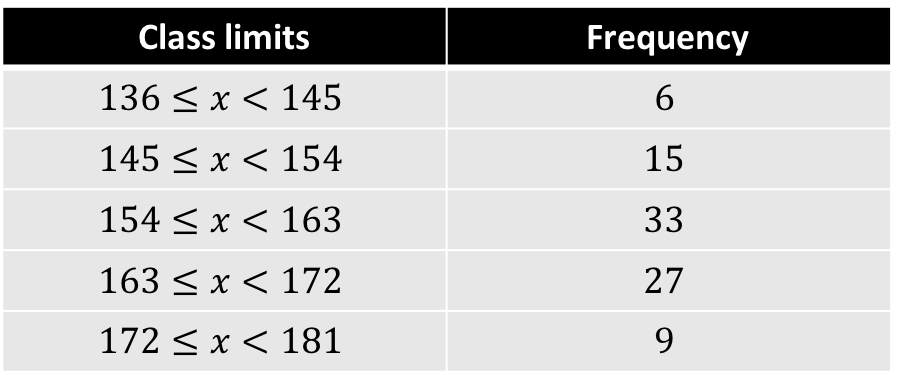Table 2: Frequency distribution of the heights (in cm) of a group of $\scriptsize 90$ students

We follow a similar process as with the bar graph to produce the histogram. We plot our classes along the x-axis and the number or frequency of each class along the y-axis. Since there are five classes, the histogram will have five rectangles. The base of each rectangle is defined by its class. The height of each rectangle is determined by its frequency.

Figure 8 shows the completed histogram for this data. In this case, small labels have been placed above each bar to indicate its height. However, these are not essential.### Example 2.1

A group of cyclists calculated the total number of kilometres they each cycled in a given week. The histogram describes the data they collected.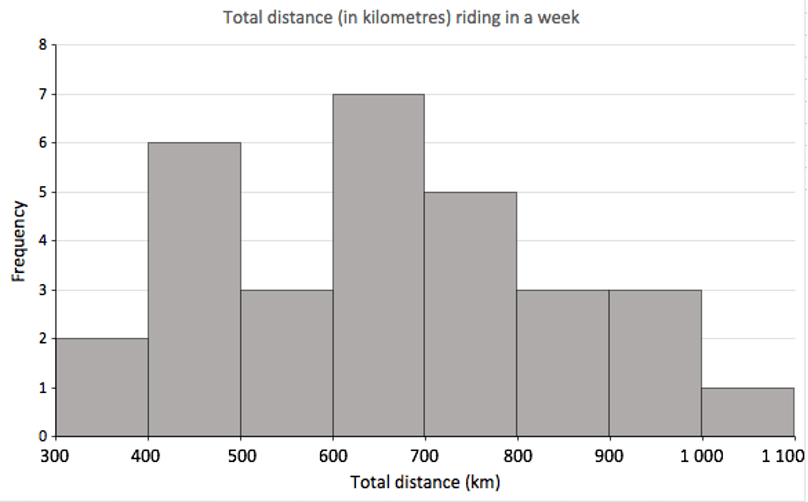1. How many cyclists completed between $\scriptsize 500$ and $\scriptsize 600$ kilometres?
2. How many cyclists completed $\scriptsize 500\ \text{km}$ or more?
3. What percentage of the cyclists completed $\scriptsize 500\ \text{km}$ or more?
4. How many cyclists completed between $\scriptsize 300$ and $\scriptsize 700$ kilometres?
5. What would you say the mean number of kilometres completed by this group of cyclists is?

Solution

1. Three cyclists completed between $\scriptsize 500$ and $\scriptsize 600$ kilometres.
2. We need to add up all the cyclists that completed between $\scriptsize 500$ and $\scriptsize 1\ 100$ kilometres. This is $\scriptsize 3+7+5+3+3+1=22$.
3. To calculate the percentage of cyclists who completed $\scriptsize 500\ \text{km}$ or more we need also know the total number of cyclists. We need to add the heights of all the bars: $\scriptsize 2+6+3+7+5+3+3+1=30$.
The percentage of cyclists who completed $\scriptsize 500\ \text{km}$ or more was $\scriptsize \displaystyle \frac{{22}}{{30}}\times 100=73.33\%$.
4. $\scriptsize 2+6+3+7=18$ cyclists completed between $\scriptsize 300$ and $\scriptsize 700$ kilometres?
5. The highest bar is the class $\scriptsize 600\le x \lt 700$. This bar is also more or less in the middle of the distribution. Therefore, the mean is likely to be within this class. We can approximate the overall mean as the mean of this class i.e. $\scriptsize \bar{x}=\displaystyle \frac{{600+700}}{2}=650\ \text{km}$.### Exercise 2.2

The mass of loaves of bread coming off the production line were measured. The results are shown in the histogram below.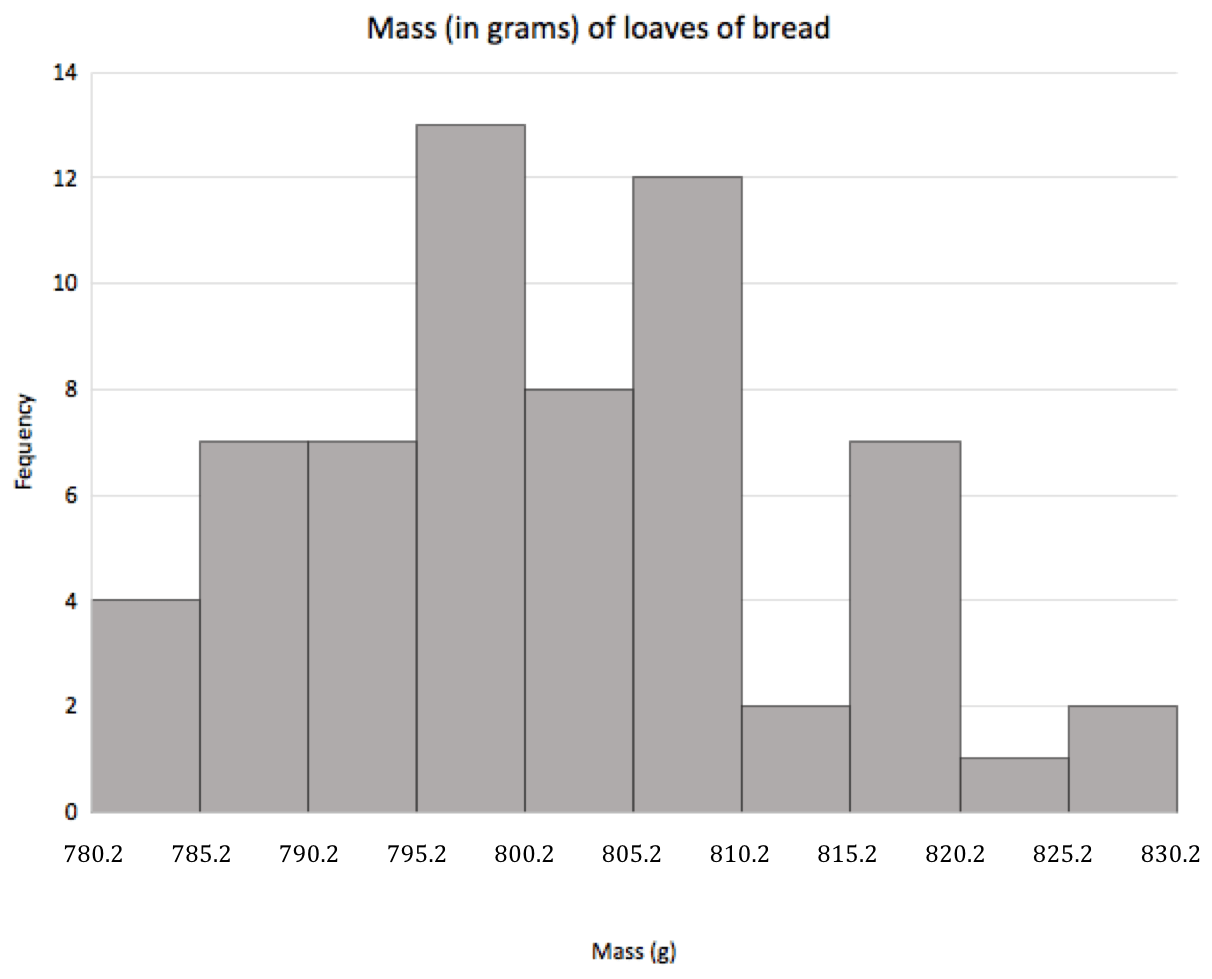1. How many loaves of bread were measured in total?
2. How many loaves of bread had a mass greater than $\scriptsize 785.2\ \text{g}$ but less than or equal to $\scriptsize 800.2\ \text{g}$?
3. What percentage of the loaves had a mass greater than $\scriptsize 810.2\ \text{g}$?

The full solutions are at the end of the unit.

## Summary

In this unit you have learnt the following:

• What the difference is between a bar graph and a histogram.
• How to draw a bar graph.
• How to draw a compound bar graph.
• How to draw a histogram.

# Unit 2: Assessment

#### Suggested time to complete: 30 minutes

1. A survey was conducted among a group of commuters. One of the questions was what cell phone network they preferred. The results are shown below.1. Create a tally table or frequency distribution of this data.
2. Construct a bar graph of this data.
3. What was the most popular cell phone network?
4. What was the least popular cell phone network?
2. The results of a survey asking families how much they spent on food in the previous week was conducted. The results are shown below.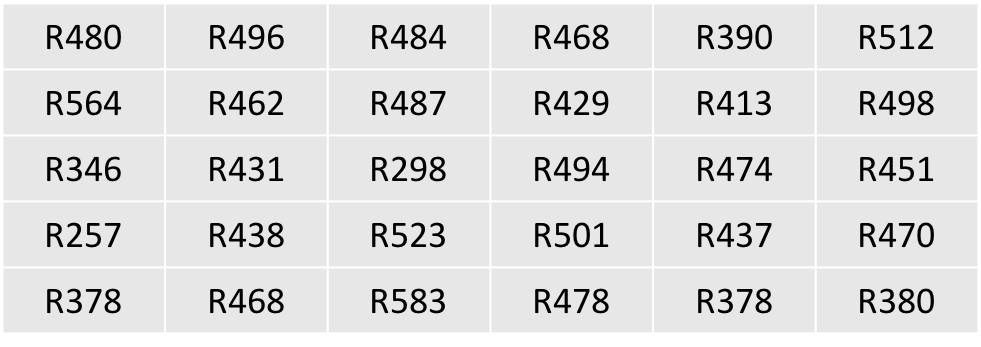1. Complete the following frequency distribution for the data.2. Use this completed frequency distribution to construct a histogram.
1. How many families spent less than $\scriptsize \text{R}312$ on food in the previous week?
2. How many families spent $\scriptsize \text{R}477$ or more on food in the previous week?
3.  How many families spent between $\scriptsize \text{R}367$ and $\scriptsize \text{R}532$ on food in the previous week?
4. What percentage of families spent $\scriptsize \text{R}532$ or more on food in the previous week?

The full solutions are at the end of the unit.

# Unit 2: Solutions

### Exercise 2.1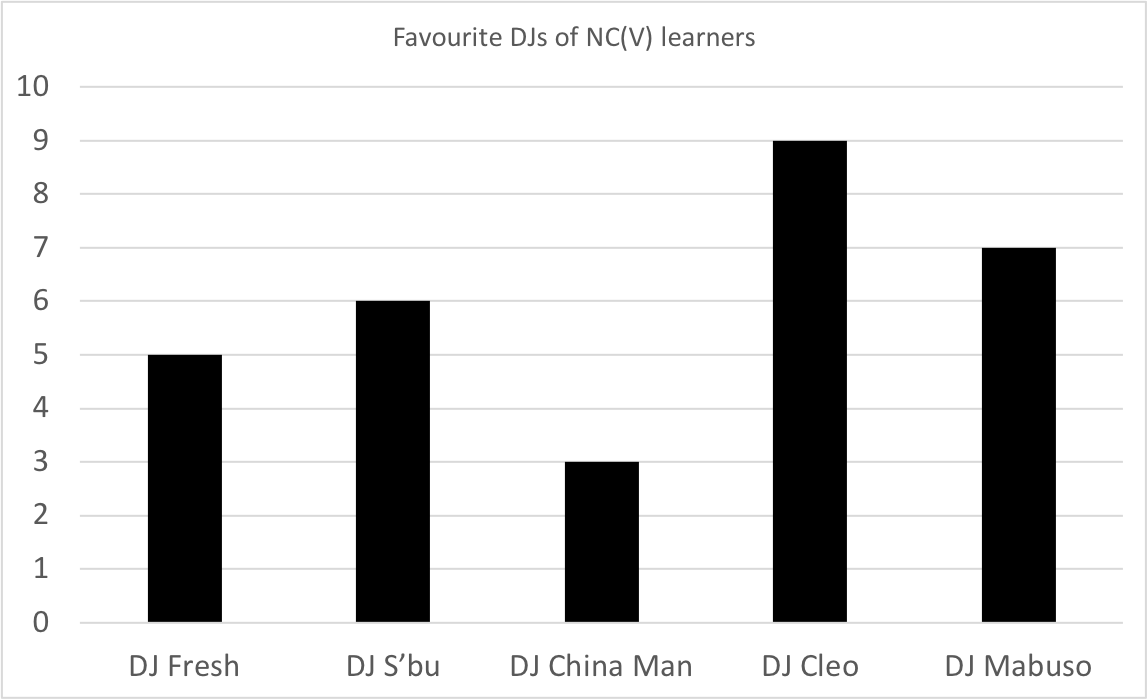Back to Exercise 2.1

### Exercise 2.21. $\scriptsize 63$ loaves were measured in total
2. $\scriptsize 7+7+13=27$
3. $\scriptsize \displaystyle \frac{{2+7+1+2}}{{63}}\times 100=\displaystyle \frac{{12}}{{63}}\times 100=19.05\%$ of the loaves had a mass greater than $\scriptsize 810.2\ \text{g}$.

Back to Exercise 2.2

### Unit 2: Assessment

1. .
1. .2. .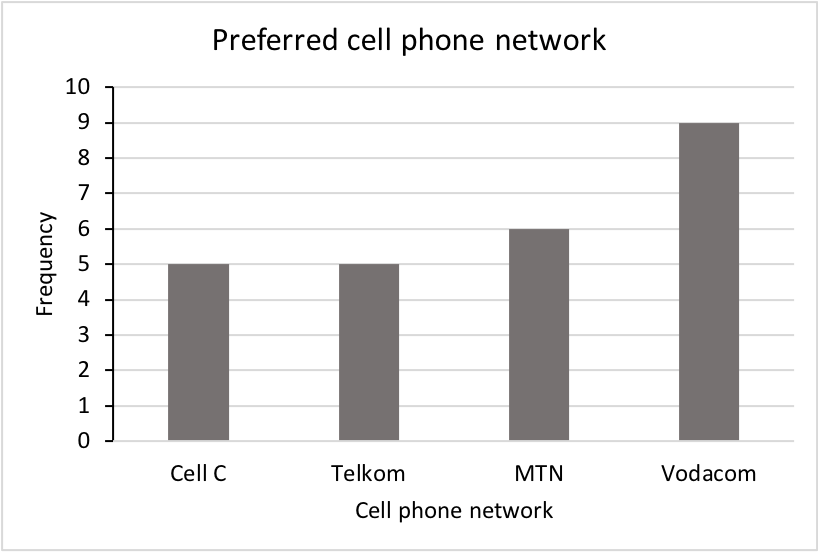3. Vodacom
4. Both Cell C and Telkom were the least popular.
2. .
1. .2. .3. .
1. Two families.
2. $\scriptsize 12$ families.
3. $\scriptsize 25$ families.
4. Total families is $\scriptsize 30$. Percentage of families spending $\scriptsize \text{R}532$ or more is $\scriptsize \displaystyle \frac{2}{{30}}\times 100=6.67\%$

Back to Unit 2: Assessment